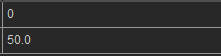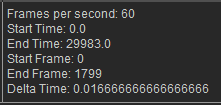# IC Python API:Handling Time

Main article: RL Python Samples.

Handling time and animations is a common operation in iClone. As a developer, you will likely come across the need to manipulate the timeline in code at some point in your projects. This article will show you how to wrap common timeline functions into one convenient class making it a super tool when it comes to timeline management. You can take the same concept to other areas of scripting, such as making custom classes for commonly accessed functions in other areas of the application.

## Required Modules

The only module we'll need is the fundamental Reallusion Python module.

```import RLPy
```

## Initializing the Timeline Class

Let's create a custom Timeline class and populate it with useful instance variables.

```class Timeline():

def __init__(self):
self.__fps = RLPy.RGlobal.GetFps()
self.__start_time = RLPy.RGlobal.GetStartTime()
self.__end_time = RLPy.RGlobal.GetEndTime()
self.__start_frame = RLPy.RTime.GetFrameIndex(self.__start_time, self.__fps)
self.__end_frame = RLPy.RTime.GetFrameIndex(self.__end_time, self.__fps)
self.__delta_time = 1 / self.__fps  # The completion time in seconds sinces the last frame
```

Notice that the instance variables are prefixed with double "_" underscores. This is because in Python, there is no existence of "Private" instance variables which cannot be accessed except inside an object. However, a convention is being followed by most Python code and coders, such that names prefixed with underscores should be treated as non-public parts of the API and Python code. Use of underscores abide by the following conventions:

• Single leading "_" underscore means you shouldn't access this variable or method because it's not part of the API. However, although discouraged, you are not technically prevented from access.
• Double leading "__" underscores emulate private variables by having Python mangle the names so that they are not easily visible to code outside of the class that contains them. Although, you can technically get around this protection.

## Adding Member Properties and Functions

Now that we have initialized the private variables, we still can not access them. In order to be useful, we'll need to add some read only member properties and member functions to the Timeline class:

```    @property
def start_frame(self):
return self.__start_frame

@property
def end_frame(self):
return self.__end_frame

@property
def delta_time(self):
return self.__delta_time

@property
def start_time(self):
return self.__start_time

@property
def end_time(self):
return self.__end_time

def IndexedFrameTime(self, frame_index):
return RLPy.RTime.IndexedFrameTime(frame_index, self.__fps)
```
```The @Property decorator turns a method into a "getter" for read-only attributes with the same name.  It can also set a custom docstring for the given method.
```Print out for the start frame and time at the 3rd frame in milliseconds.

Now we can query the Timeline member properties, for example:

```timeline = Timeline()
print(timeline.start_frame) # Get the start frame
print(timeline.IndexedFrameTime(3).GetValue()) # Get the time in ms at the 3rd frame
```

## Modifying Built-in FunctionsBy modifying the __str__ built-in function, we can provide a more useful and verbose print-out

We can also take advantage of Python's built-in functions like __str__ to customize the string representation of the Timeline object. For example, if we want to verbose print out of the private member variables, we can add the following code to the class:

```    def __str__(self):
return 'Frames per second: {}\nStart Time: {}\nEnd Time: {}\nStart Frame: {}\nEnd Frame: {}\nDelta Time: {}'.format(
self.__fps, self.__start_time.GetValue(), self.__end_time.GetValue(), self.__start_frame, self.__end_frame, self.__delta_time)
```

Now when we print the Timeline instance object, we get verbose internal details:

```timeline = Timeline()
print(timeline)
```

## Everything Put Together

You can copy and paste the following code into a PY file and load it into iClone via Script > Load Python.

```import RLPy

class Timeline():

def __init__(self):
self.__fps = RLPy.RGlobal.GetFps()
self.__start_time = RLPy.RGlobal.GetStartTime()
self.__end_time = RLPy.RGlobal.GetEndTime()
self.__start_frame = RLPy.RTime.GetFrameIndex(self.__start_time, self.__fps)
self.__end_frame = RLPy.RTime.GetFrameIndex(self.__end_time, self.__fps)
self.__delta_time = 1 / self.__fps  # The completion time in seconds sinces the last frame

def __str__(self):
return 'Frames per second: {}\nStart Time: {}\nEnd Time: {}\nStart Frame: {}\nEnd Frame: {}\nDelta Time: {}'.format(
self.__fps, self.__start_time.GetValue(), self.__end_time.GetValue(), self.__start_frame, self.__end_frame, self.__delta_time)

@property
def start_frame(self):
return self.__start_frame

@property
def end_frame(self):
return self.__end_frame

@property
def delta_time(self):
return self.__delta_time

@property
def start_time(self):
return self.__start_time

@property
def end_time(self):
return self.__end_time

def IndexedFrameTime(self, frame_index):
return RLPy.RTime.IndexedFrameTime(frame_index, self.__fps)
```

## APIs Used

You can research the following references for the APIs deployed in this code.